# High School Math : How to find the length of the hypotenuse of a 45/45/90 right isosceles triangle : Pythagorean Theorem

## Example Questions

### Example Question #1 : 45/45/90 Right Isosceles Triangles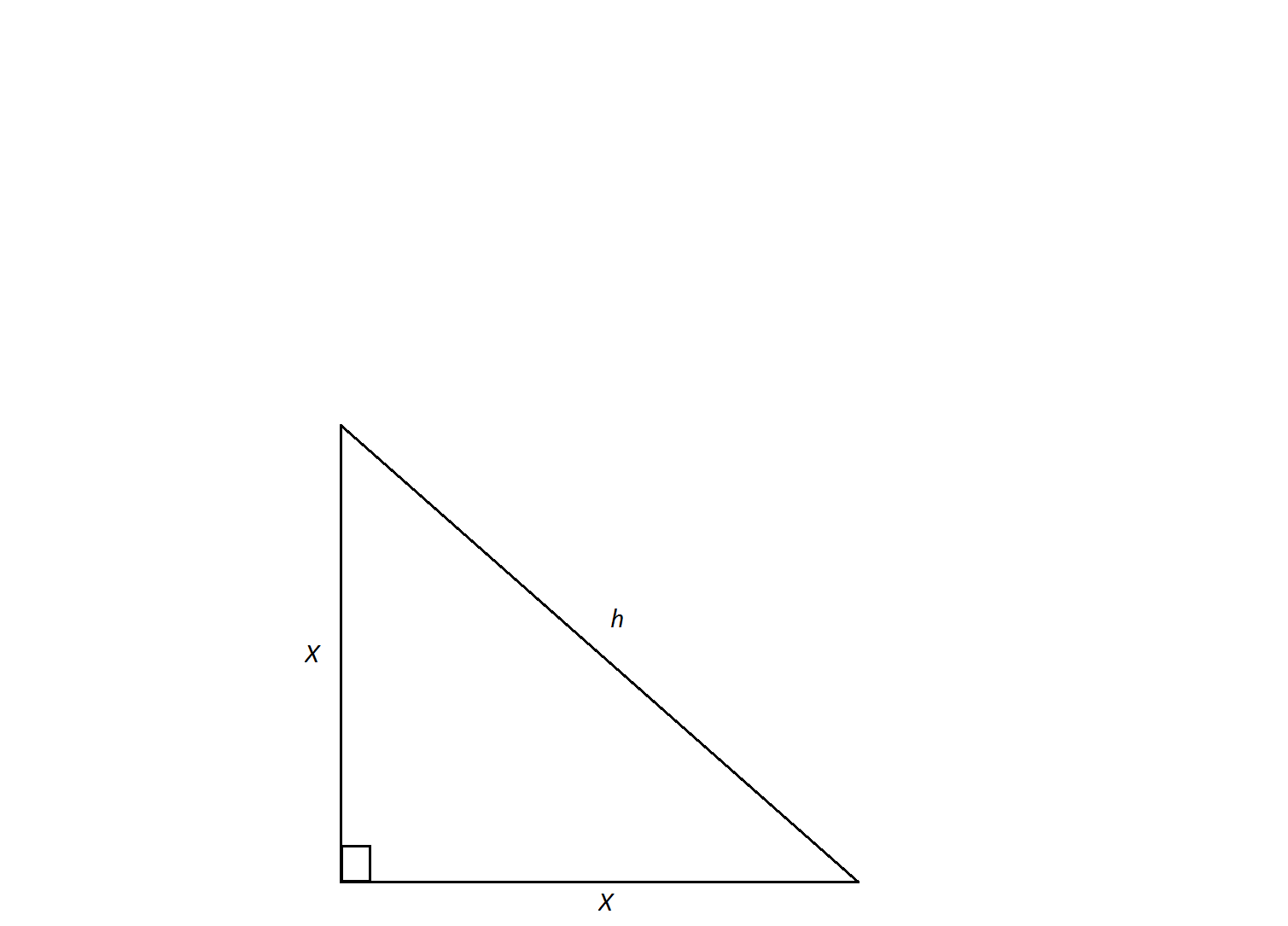In an isosceles right triangle, two sides equal. Find the length of side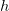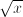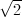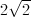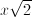Explanation:

This problem represents the definition of the side lengths of an isosceles right triangle.  By definition the sides equal, and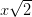.  However, if you did not remember this definition one can also find the length of the side using the Pythagorean theorem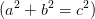.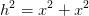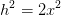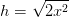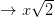### Example Question #2 : 45/45/90 Right Isosceles Triangles

ABCD is a square whose side isunits.  Find the length of diagonal AC.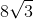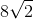Explanation:

To find the length of the diagonal, given two sides of the square, we can create two equal triangles from the square. The diagonal line splits the right angles of the square in half, creating two triangles with the angles of,, anddegrees. This type of triangle is a special right triangle, with the relationship between the side opposite thedegree angles serving as x, and the side opposite thedegree angle serving as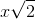.

Appyling this, if we plugin forwe get that the side opposite the right angle (aka the diagonal) is### Example Question #3 : 45/45/90 Right Isosceles Triangles

The area of a square is. Find the length of the diagonal of the square.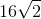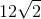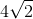If the area of the square is, we know that each side of the square is, because the area of a square is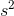.
Then, the diagonal creates two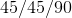special right triangles. Knowing that the sides =, we can find that the hypotenuse (aka diagonal) is